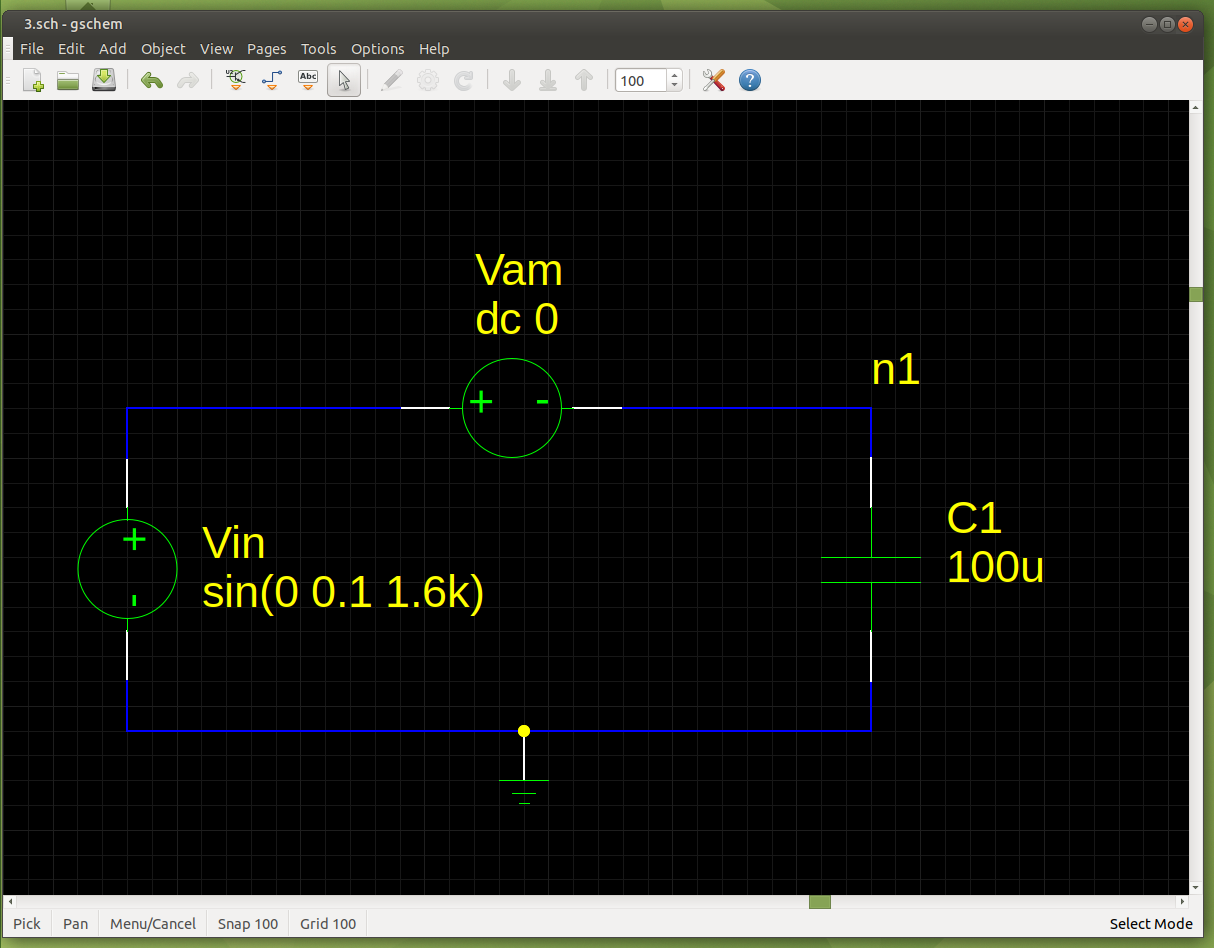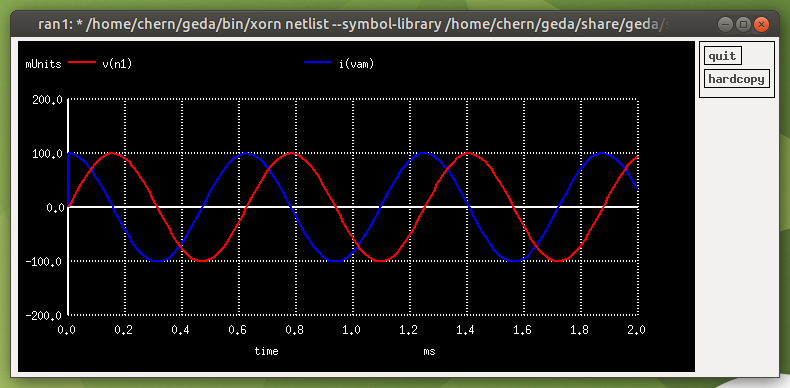# AC Capacitor

Let's use this circuit to see the current through a capacitor as the voltage across it changes:We want the peak current through the capacitor to be equal to the peak voltage across it. Using Ohm's Law, we can simply divide Vin (0.1V) by 1Ω to get 0.1A. The impedance of the circuit is equal to the reactance plus resistance. For simplicity, I have not used a resistor in the circuit so the impedance is just the reactance: Xc = 1/(2*Pi*f*C). To find the value of the capacitor, we rearrange the formula: C = 1/(2*Pi*f*Xc). We want Xc to be 1, so C = 1/(2*Pi*f*1). f = 1.6kHz, so C = 1/(2*Pi*1600*1). We get an answer of about 99.472uF. Let's round it up to the nearest E24 preferred value, 100uF.

Transient Analysis:

`tran 0.01m 2m`
`plot v(n1) i(vam)`We can see that as the voltage (red) is at its peak (zero change), the current (blue) is 0. When the voltage is at its steepest slope (maximum change), the current is at its maximum (about ±0.1A). The relationship between the voltage and current is one from calculus, i = C(dv/dt). We can also see that the voltage lags behind the current by 90 degrees (the voltage wave is -90° out of phase with the current wave).